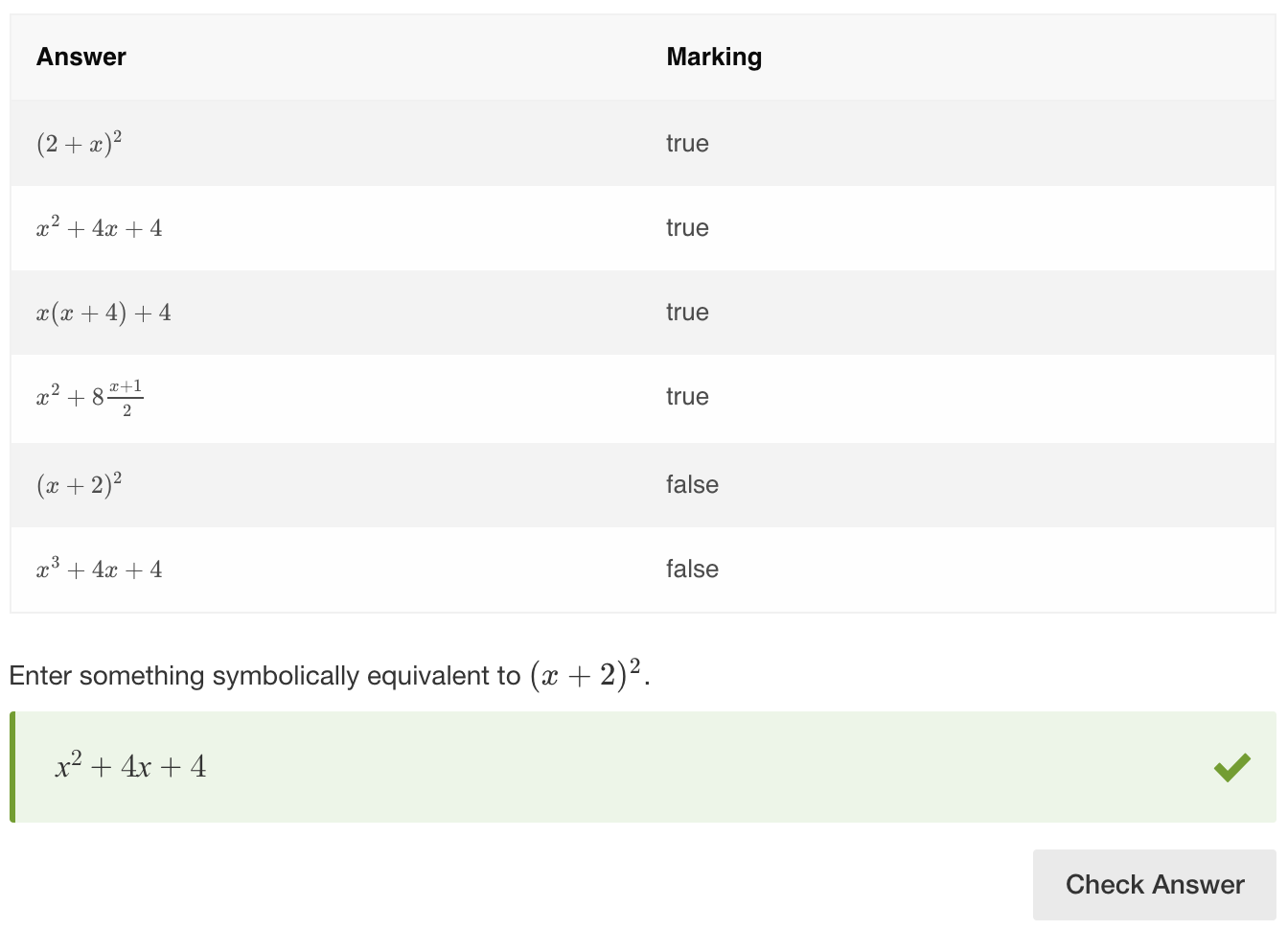# equivSymbolic

## Overview

The equivSymbolic method checks that the value entered by the learner is mathematically equivalent to the value that the Author has set in the validation, even if they are in different forms.

Use equivSymbolic when working with expressions where order or form is not important. For example, if the task is to multiply 3 and 2, any response that is mathematically equal to 6 will be considered as correct, such as 6/1, 12/2, 3+3, 6.0.

equivSymbolic accepts a wide range of inputs including decimals, fractions, variables, percentages, trigonometric, logarithmic, and exponential expressions.

• Significant decimal places
This option specifies the number of significant decimal places used to compare the learner's response and the Author's validation value. The maximum and default value is 10.
• Allow interval
This option must be enabled when the learner is expected to insert interval notation in the response area, ie  ‘[1, 4)’. Without this option enabled, the response may not validate correctly.
• Ignore text
This refers to LaTeX text only, and when enabled the scoring engine will ignore any LaTeX text the learner enters in the response area.
• Compare sides
With this option enabled, when comparing two equations the math engine will compare the corresponding sides of each equation rather than the whole equations.
• Treat 'e' as Euler's number
This option treats the variable 'e' as Euler's number. This overrides the default interpretation of 'e' as scientific notation.
• Allow decimal marks
Authors can specify what separators can be used by the learner. From the Thousand Separator drop-down dot, comma, and/or space can be selected. From the Decimal Separator drop-down either a dot or a comma can be selected. Note that the specified thousand separators and decimal separator cannot be the same, e.g. both dot.
• Treat all letters as variables
When this option is enabled, the math engine will treat all letters as variables. This is useful in cases where you want to use letters as variables that the math engine may normally recognize as units of measurement. Here are the units of measurement we support: List of S.I and US Customary Units

## Examples

Example 1 - Basic equivSymbolic scoring

In this example, any value that is equal to 100 will be considered as correct.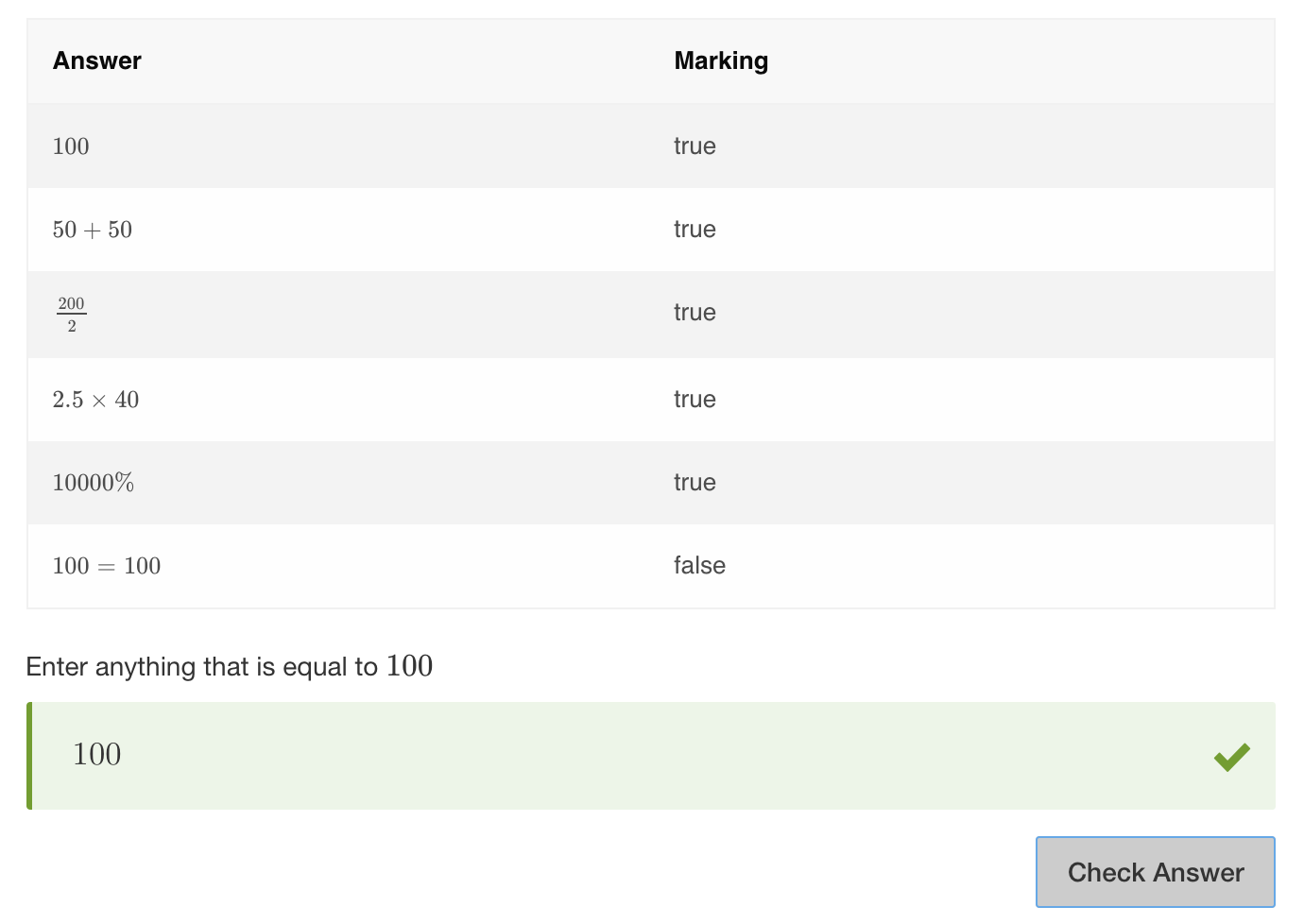Example 2 - equivSymbolic fill in the blanks

In this question, the students enter a value in the response box in order to complete the equation. Anything that is equal to 100 must be entered in the response box.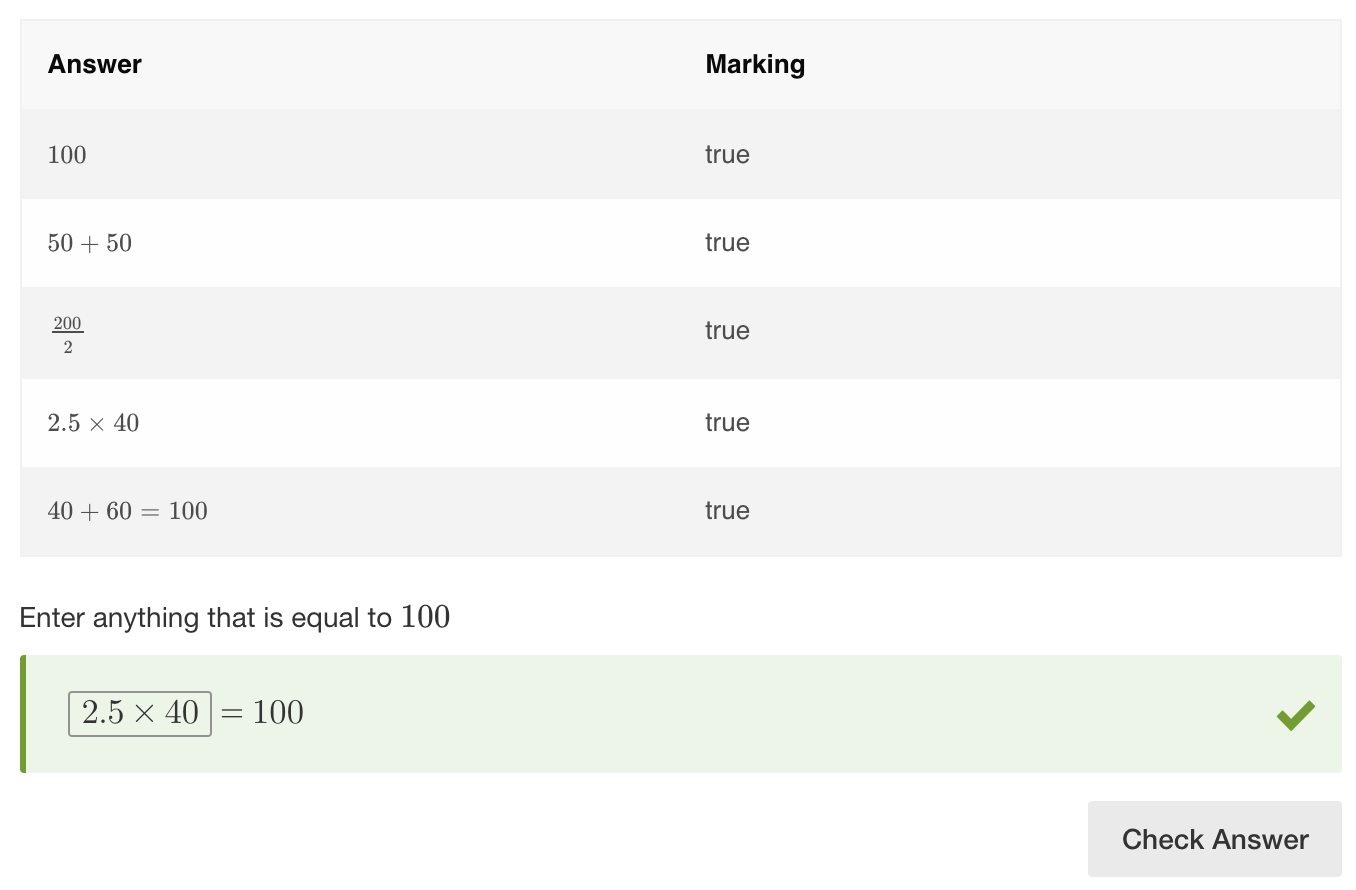Example 3 - equivSymbolic fill in the blanks

This is a similar example, only with two blank spaces that have to be filled in. Two values that are equal to 100 must be entered in the response boxes. Order is not important.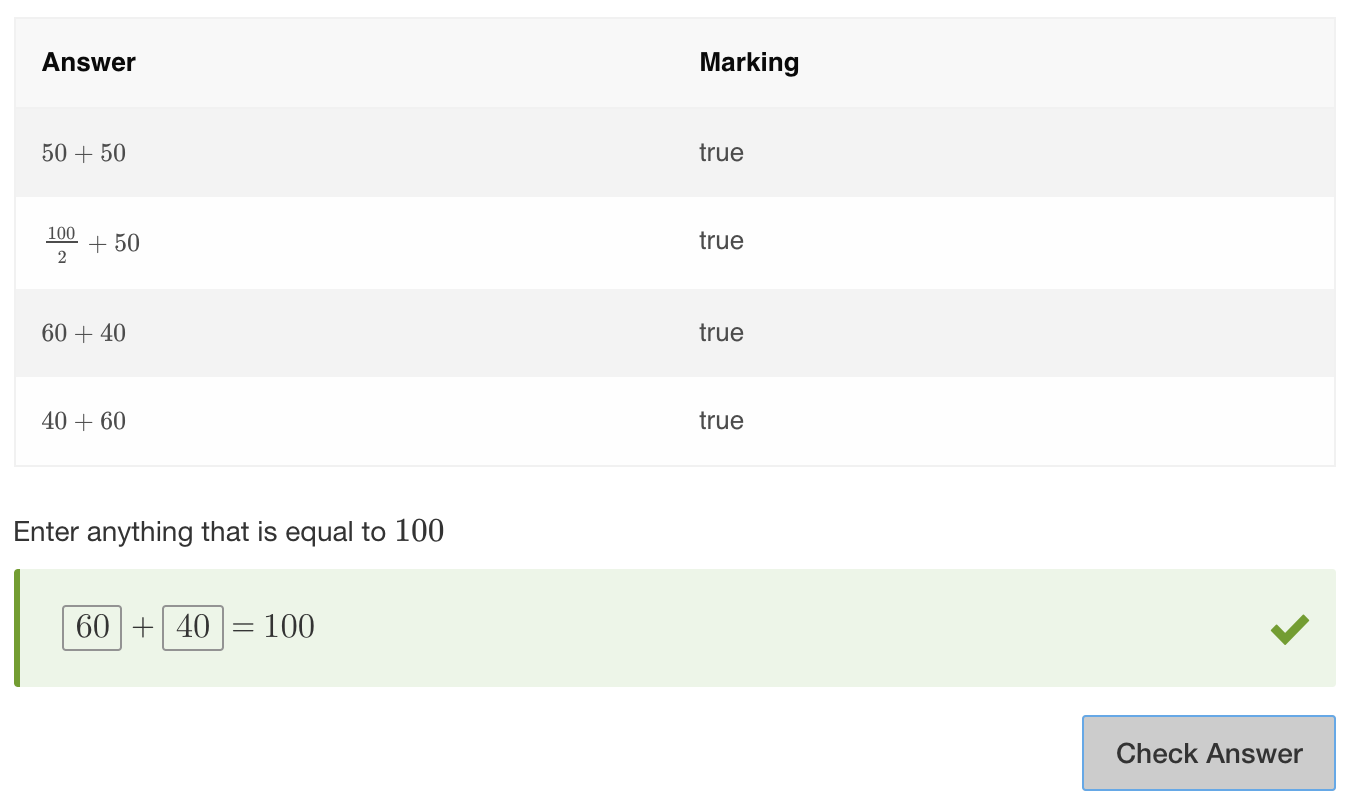Example 4 - equivSymbolic fill in the blanks

This example has 3 empty boxes. The valid response is set to 2+2=4. As neither side of the equation has a set value, it is scored as isTrue. Any equation inserted in the response boxes will be correct as long as it represents a mathematically correct equation in the form x+y=z.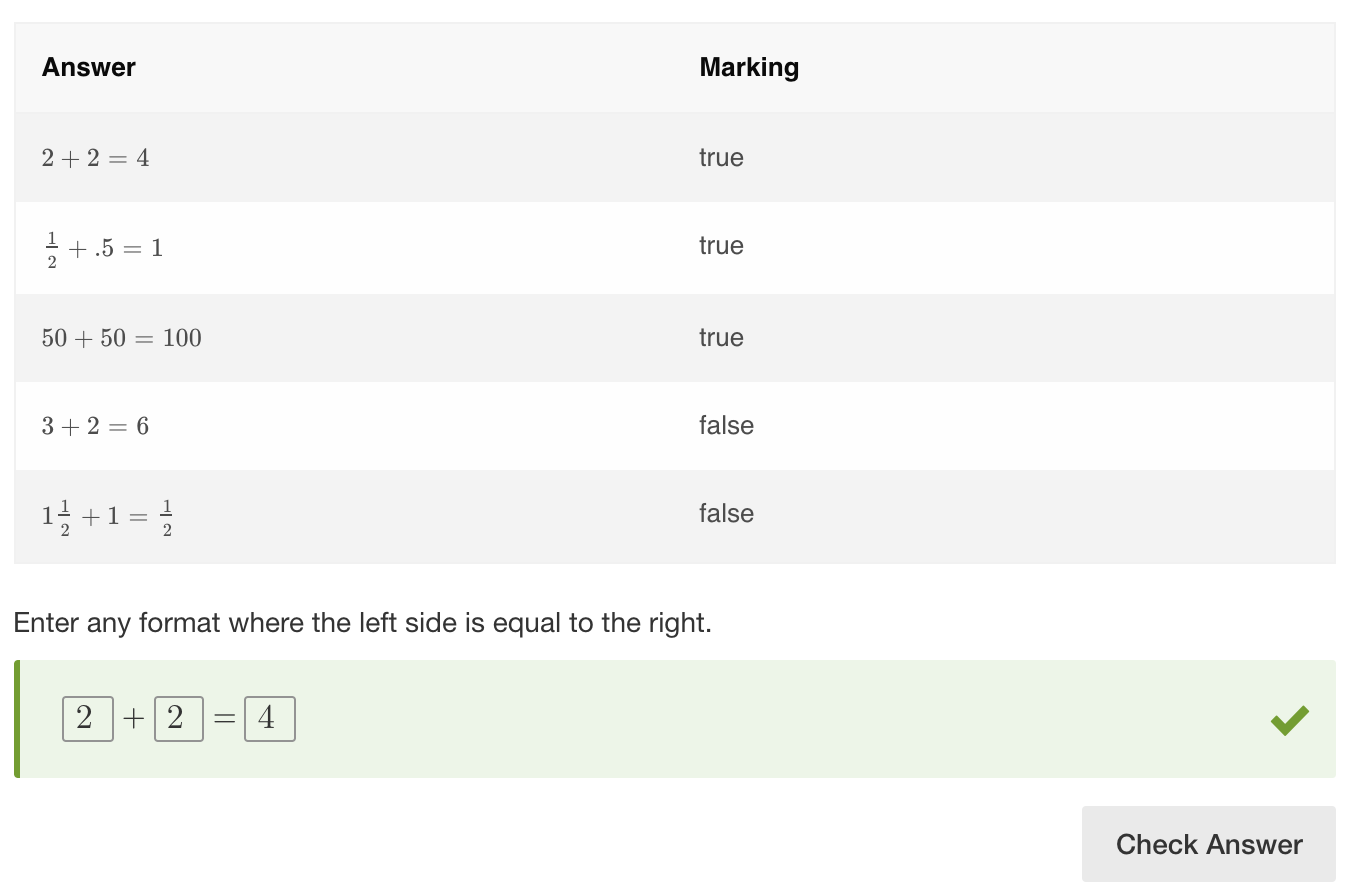Example 5 - equivSymbolic with fractions

equivSymbolic handles responses containing fractions of different types.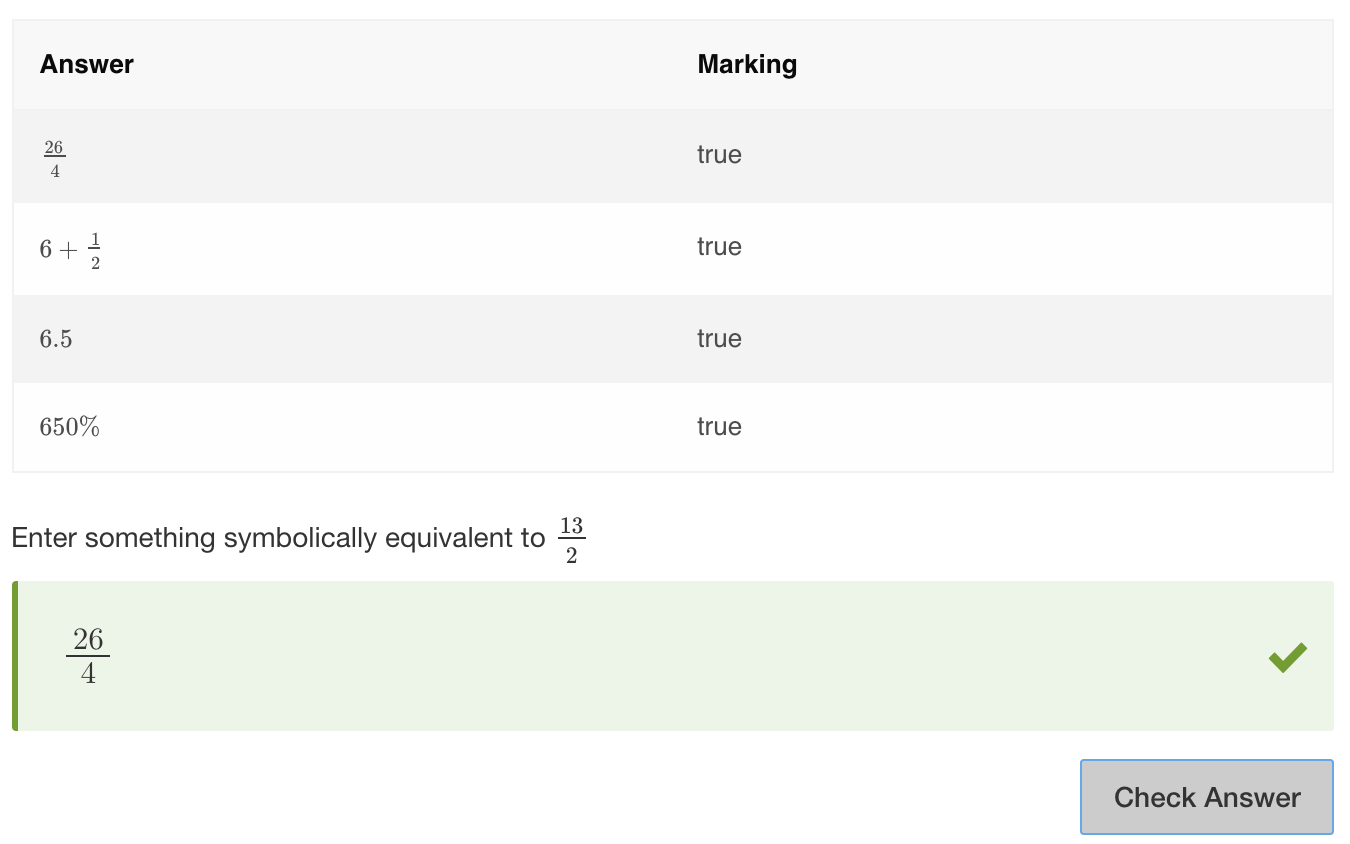Example 6 - equivSymbolic with variables

equivSymbolic can also be used with variables.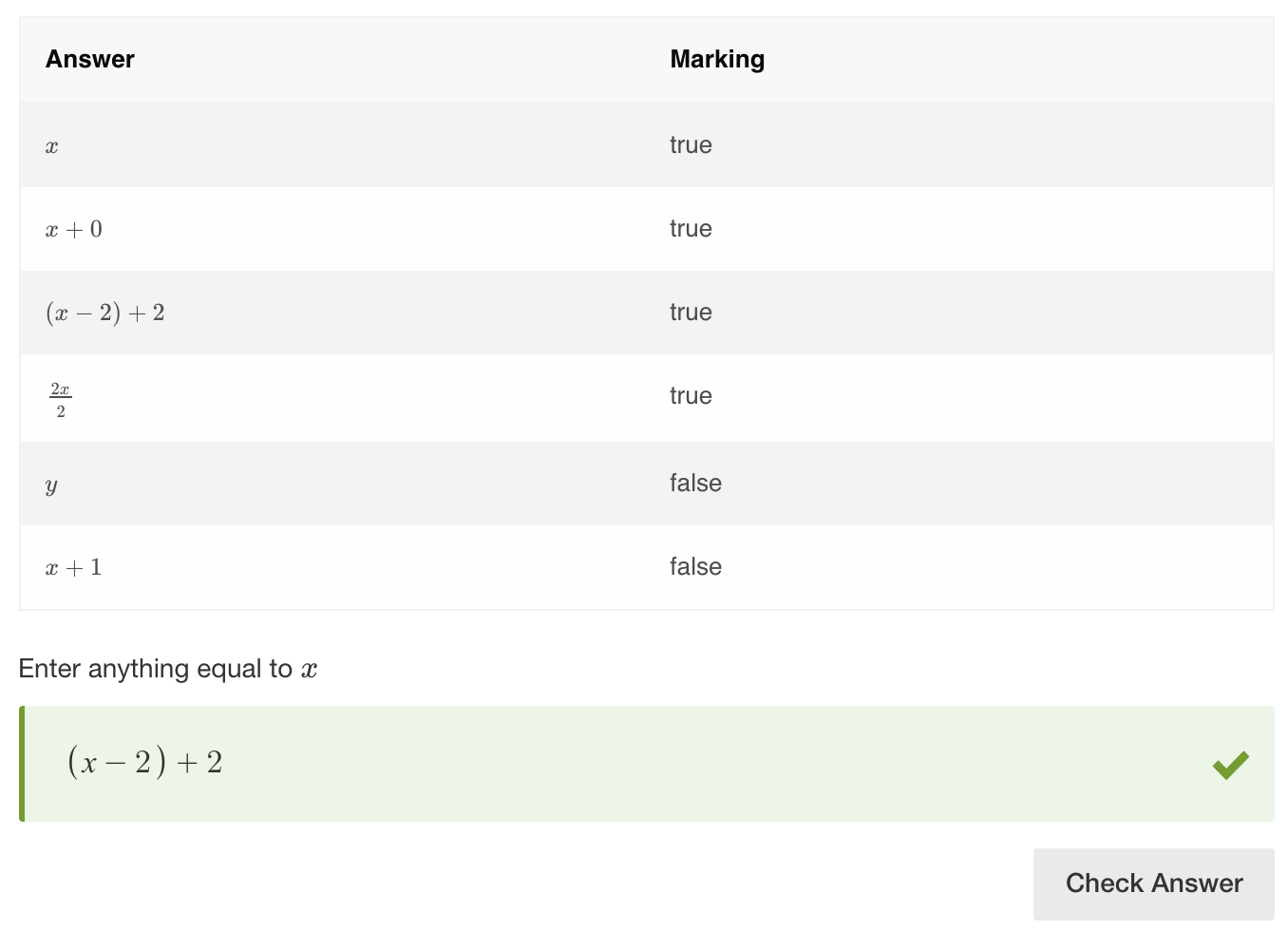Example 7 - equivSymbolic with trigonometry questions

equivSymbolic recognises trigonometric functions.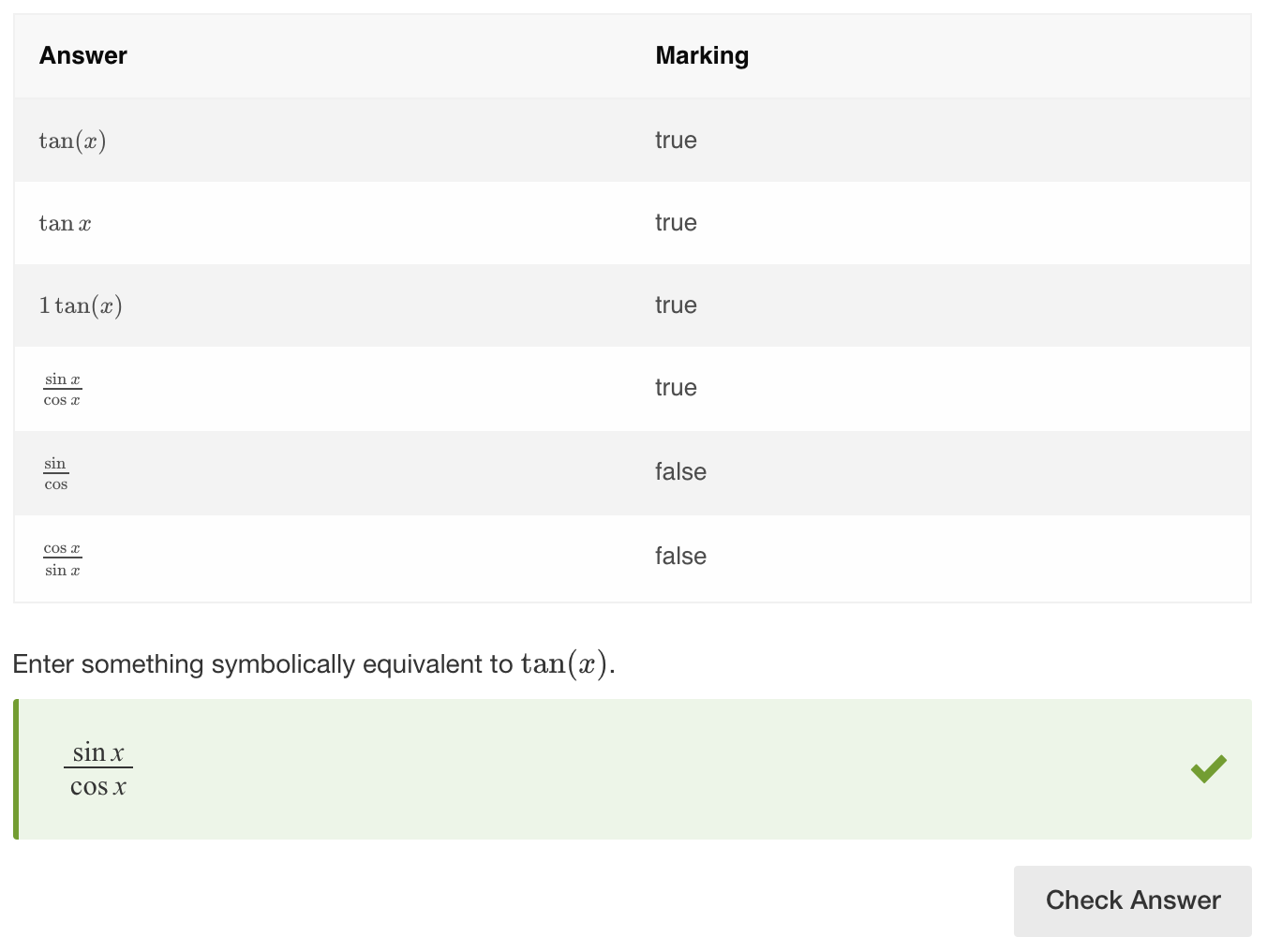Example 8 - equivSymbolic with algebraic expressions

In the example below, an expression in any form that is mathematically equivalent to the one in the stimulus will be correct.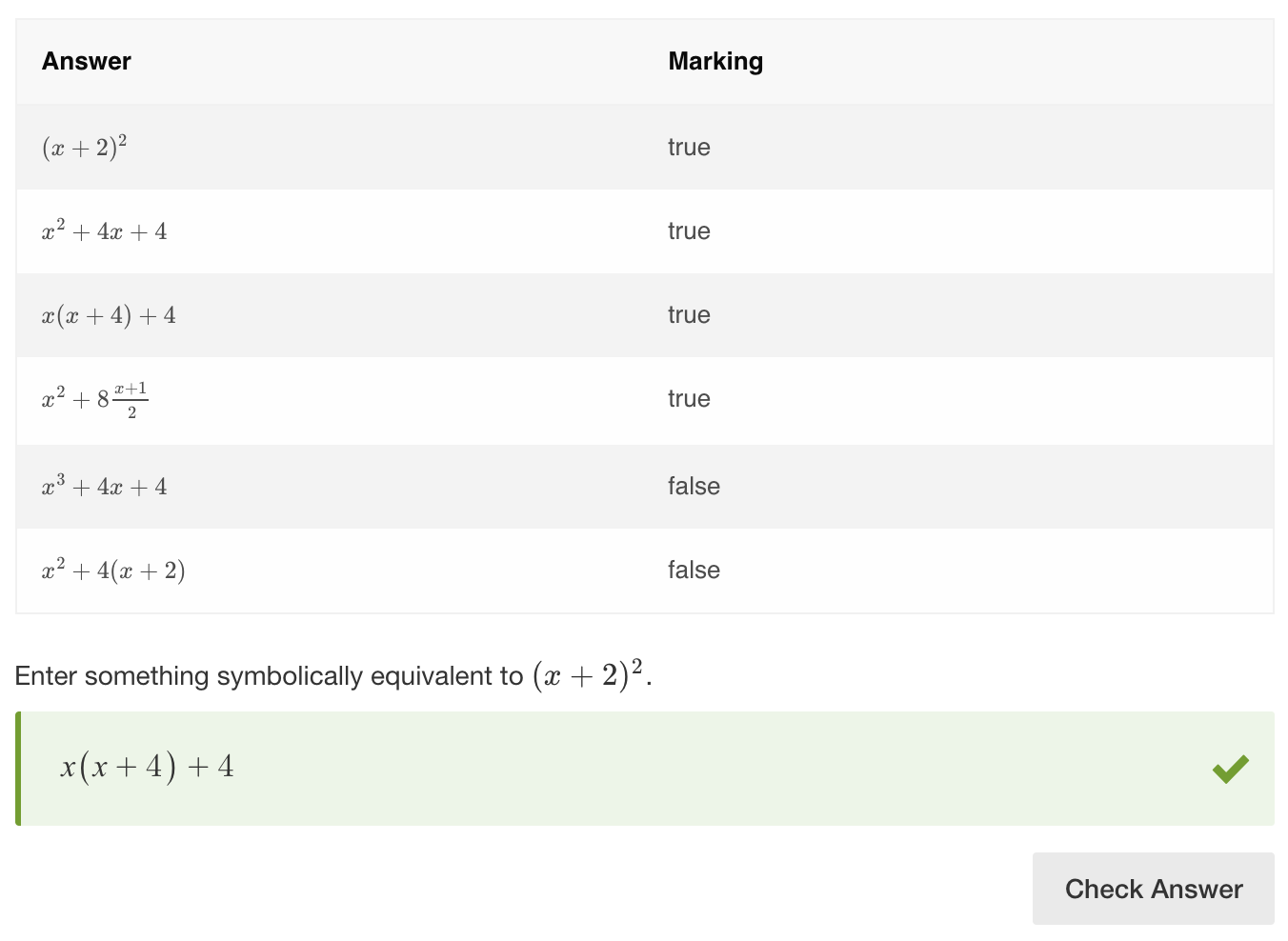Example 9 - decimal rounding

The decimal places field is used to specify a number of significant decimal places in the response. In this example, the significant decimal places option is set to 2. This way, the learner will not need to enter all the digits after the decimal point for the response to be deemed correct.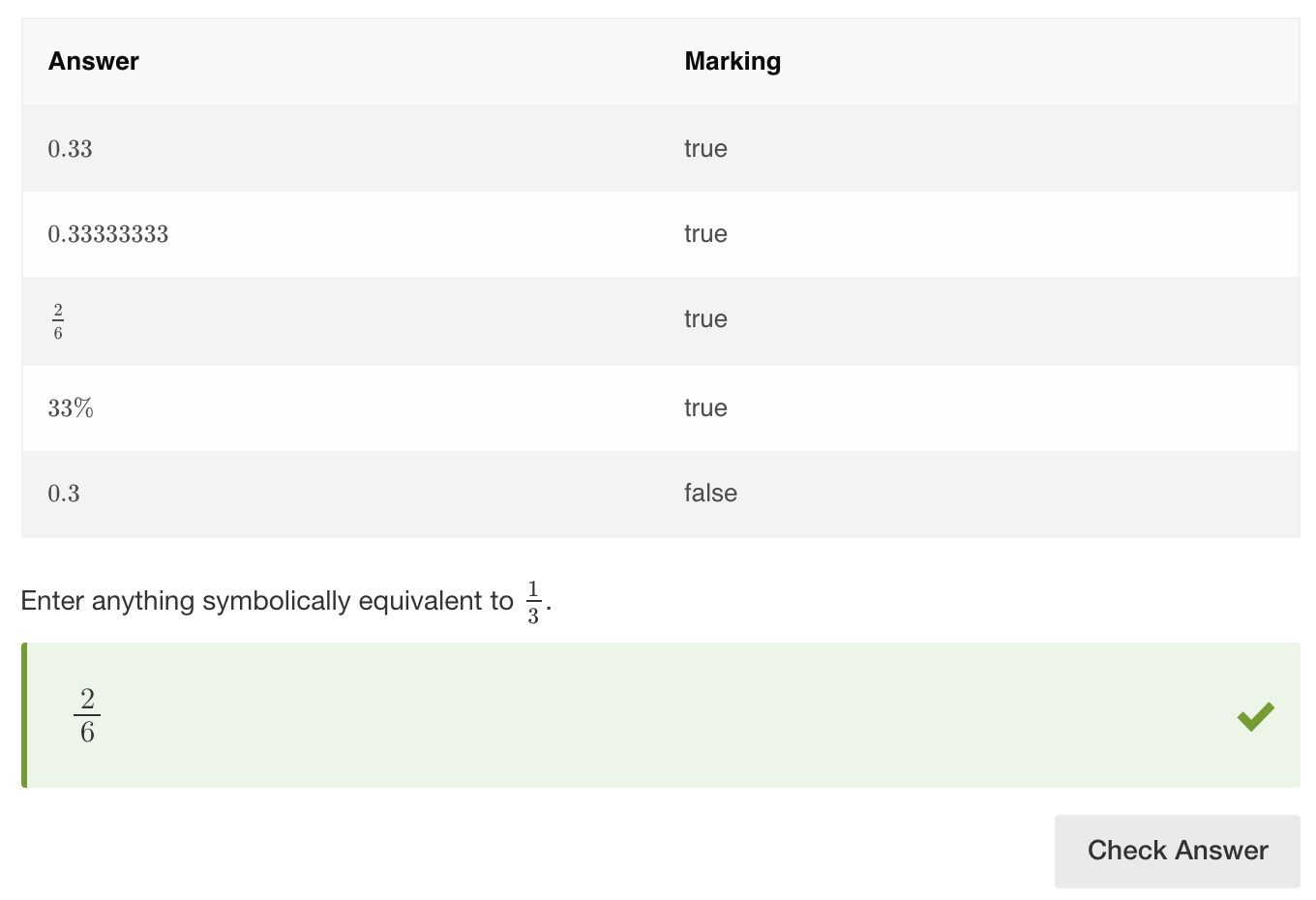Example 10 - using text blocks

You can set custom units such as ft, kg, mi, etc. as text blocks in the More options section. This means that the units will not be rendered as LaTeX.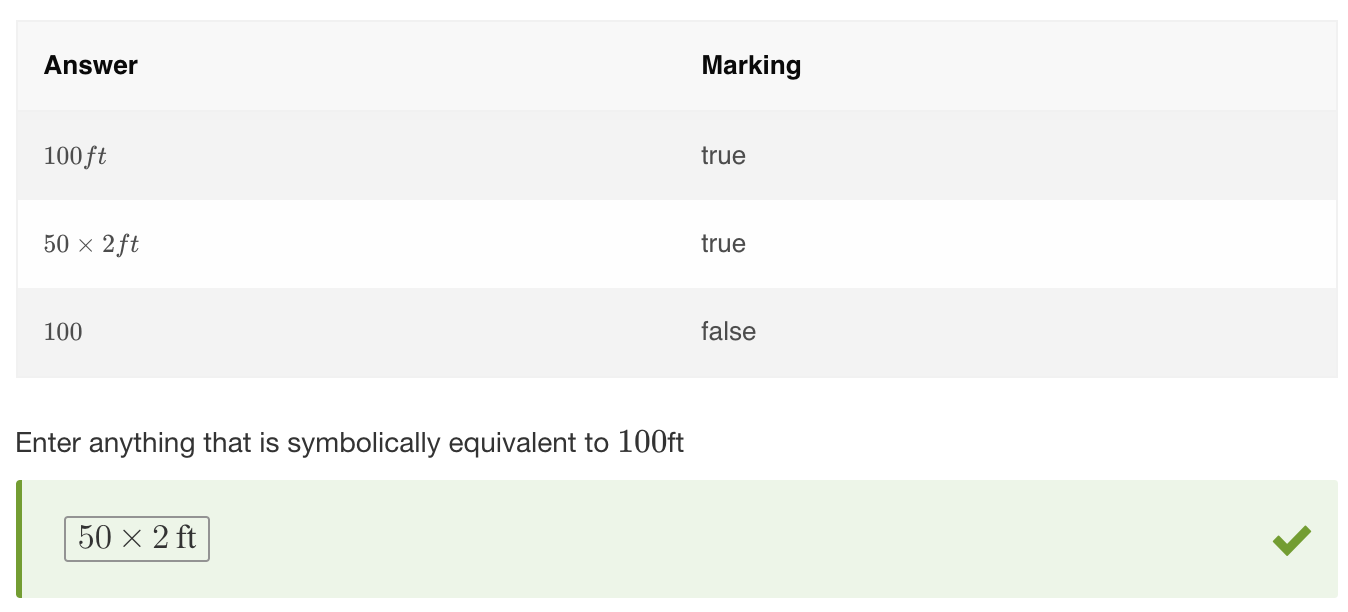Example 11 - using compare sides

Compare sides is used with open-ended questions. It ensures the equation values entered by the student give the same mathematical result and are symbolically equivalent to the equation set by the author.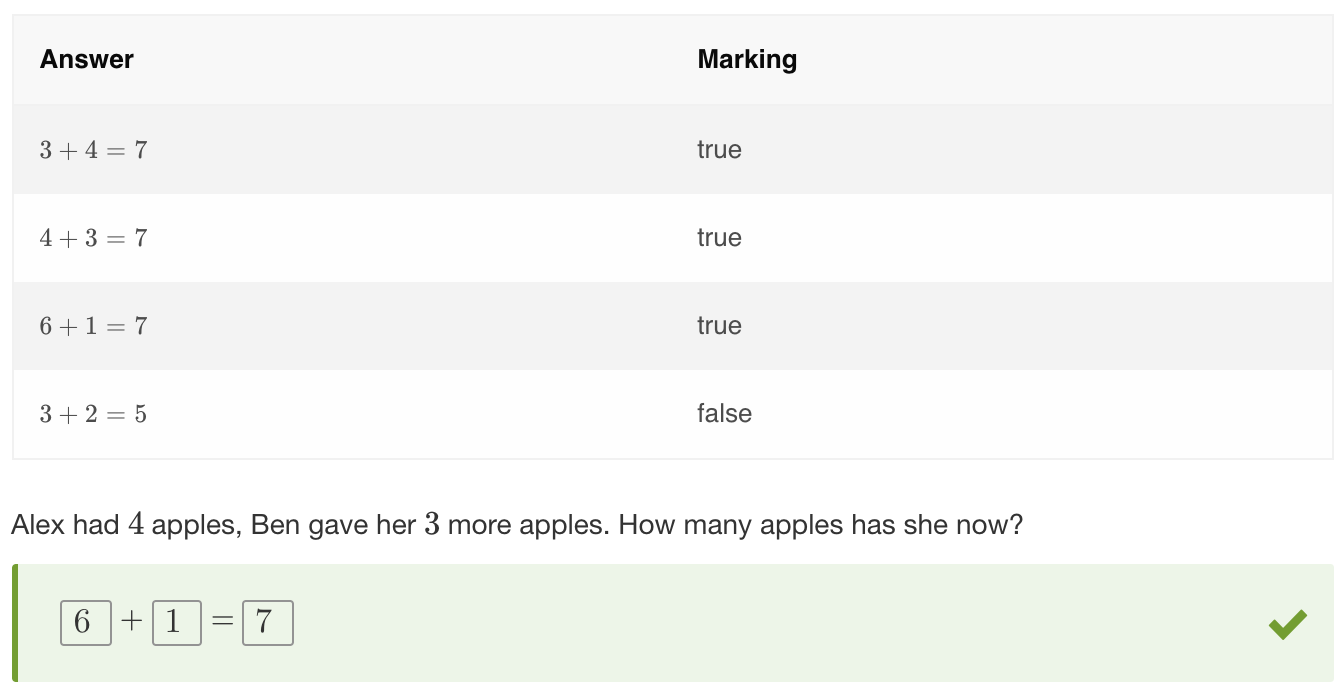## Combining methods

equivSymbolic is a very flexible method and is often used in conjunction with other methods.

Example - Excluding possible correct responses

In some cases the students might be asked to give a correct response other than the one already specified in the stimulus. Authors can set such exceptions by combining equivSymbolic and equivLiteral, then enabling the inverse result option underneath equivLiteral. Now, any symbolically equivalent equation will be correct except for the actual valid response specified in question validation.